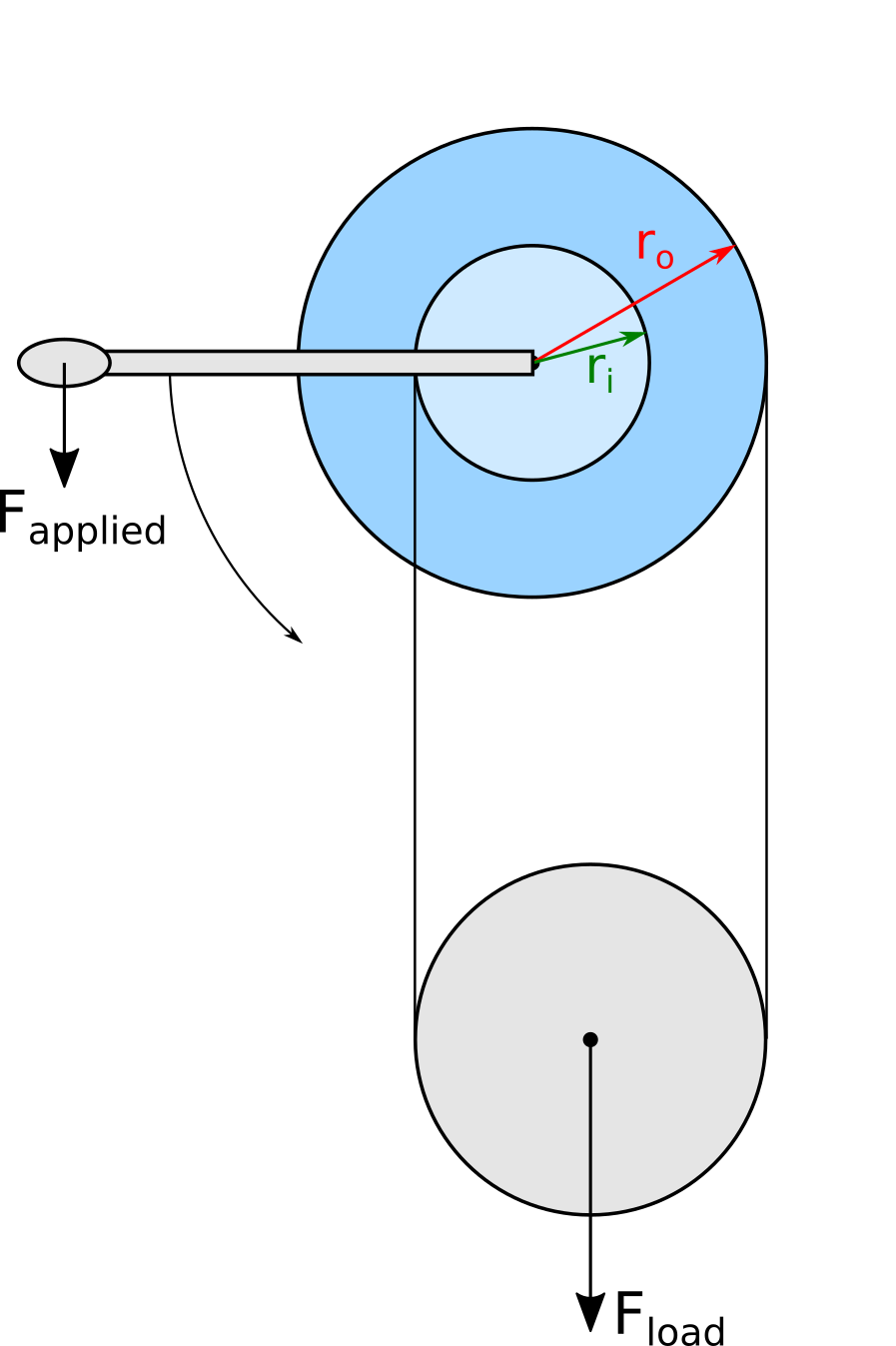# Mechanomy# Differential Winch

Continuing our MonthlyMechanism series, let's look at the differential winch.

At its core are two reels of differing diameter on the same shaft, with the ends of a single cable attached to each. Between the reels the cable circuits a pulley, onto which a load is hung. Note that reels are wound in the same direction: the cable winding on the larger reel lies on the opposite side of the shaft from the cable unwinding from the smaller reel. Turning the reels by their common shaft leads to the simultaneous winding and unwinding of cable from the reels, though at differing rates from their differing diameters. These differing rates slowly change the length of cable between the reels, raising the load if the loop length is decreasing or lowering it when increasing.The differential winch uses two reels of differing diameter and a pulley to create a large mechanical advantage. Taking the end-view and summing moments about the shaft, the radii of the larger and smaller reels can be used to find the torque-load relationship as:

$T_{applied} = \frac{F_{load}}{2} (r_{outer} - r_{inner})$

Also needed is the change in load height per crank revolution:

$\frac{\Delta h }{\theta} = ro - ri$

Users generally want to know how 'hard' it will be to raise a given load, and as designers we want to understand how a differential winch compares to other options. Assuming crank operation, how hard to raise a load can be expressed as the ratio between the force applied to the crank handle and the weight of the load, with lower ratios being easier:

$\frac{F_{applied}}{F_{load}} = \frac{r_{outer} - r_{inner}}{2r_{handle}}$

If we take the outer and handle radii to be 1, we can compare several winches: